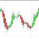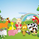1848 views
Introduction

I already estimated the least-squares moving average numerous times, one of the most elegant ways was by rescaling a linear function to the price by using the z-score, today i will propose a new smoother (FLSMA) based on the line rescaling approach and the inverse fisher transform of a scaled moving average error with the goal to provide an alternative least-squares smoother, the indicator won't use the correlation coefficient and will try to adresses problems such as overshoots and lag reduction.

Line Rescaling Method

For those who did not see my least squares moving average estimation using the line rescaling method here is a resume, we want to fit a polynomial function of degree 1 to the price by reducing the sum of squares between the price and the filter, squares is a term meaning the squared difference between the price and its estimation. The line rescaling technique work as follow :

• 1 - get the z-score of a line.
• 2 - multiply this z-score with the correlation between the price and a line.
• 3 - multiply the precedent result with the standard deviation of the price, then sum that to a simple moving average .

This process is shorter than the classical least-squares moving average method.

Z-Score Derivation And The Inverse Fisher Transform

The FLSMA will use a similar approach to the line rescaling technique but instead of using the correlation during step 2 we will use an alternative calculated from the error between the estimate and the price.

In order to do so we must use the inverse fisher transform , the inverse fisher transform can take a z-score and scale it in a range of (1,-1), it is possible to estimate the correlation with it. First lets create our modified z-score in the form of : Z = ma((y - Y)/e) where y is the price, Y our output estimate and e the moving average absolute error between the price and Y and lets call it scaled smoothed error, then apply the inverse fisher transform : r = IFT (Z) = tanh (Z), we then multiply the z-score of the line with it.

Performance

The FLSMA greatly reduce the overshoots, this mean that the maximas of abs(r) are lower than the maxima's of the absolute correlation, such case is not "bad" but we can see that the filter is not closer to the price than the LSMA during trending periods, we can assume the filter don't reduce least-squares as well as the LSMA .

The image above is the running mean of the absolute error of each the FLSMA (in red) and the LSMA (in blue), we could fix this problem by multiplying the smooth scaled error by p where p can be any number, for example :

z = sma ( src - nz (b, src ),length)/e * p where p = 2

In red the FLSMA and in blue the FLSMA with p = 2, the greater p is the less lag the FLSMA will have.

Conclusion

It could be possible to get better results than the LSMA with such design, the presented indicator use its own correlation replacement but it is possible to use anything in a range of (1,-1) to multiply the line z-score. Although the proposed filter only reduce overshoots without keeping the accuracy of the LSMA i believe the code can be useful for others.

Open-source script

In true TradingView spirit, the author of this script has published it open-source, so traders can understand and verify it. Cheers to the author! You may use it for free, but reuse of this code in a publication is governed by House Rules. You can favorite it to use it on a chart.

Want to use this script on a chart?
Patreon: https://www.patreon.com/alexgrover

You can also check out some of the indicators I made for luxalgo : https://www.tradingview.com/u/LuxAlgo/#published-scriptswaoooo alex....great job man...frankly speaking you are one of the best and genuine person in TV...thank you for all of your beautiful scripts...admire and appriciate your hardwork,,,abinash7
@abinash7, Thank you a lot for your praise and support, let me know if you have suggestions or anything i could help with :)Nice works Alexgrover; keep it up; cheer!
We will always love to seeing you creating new stuff in public =D